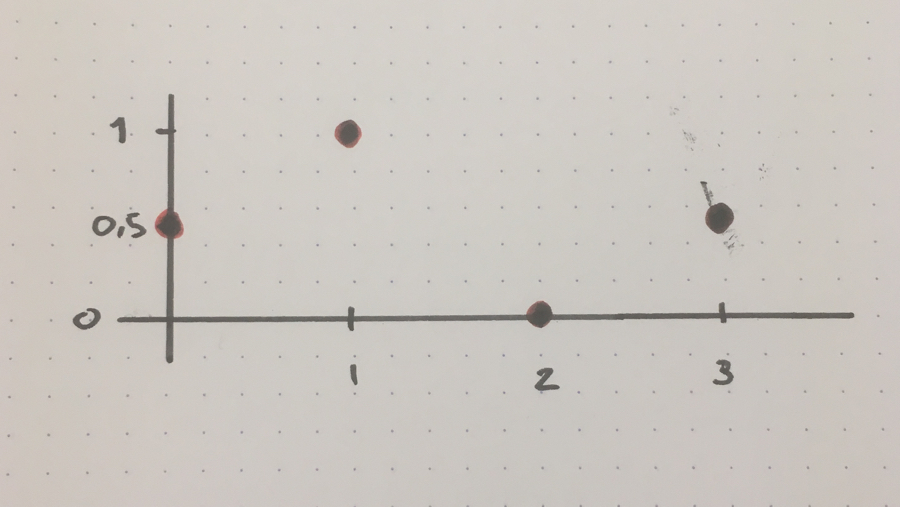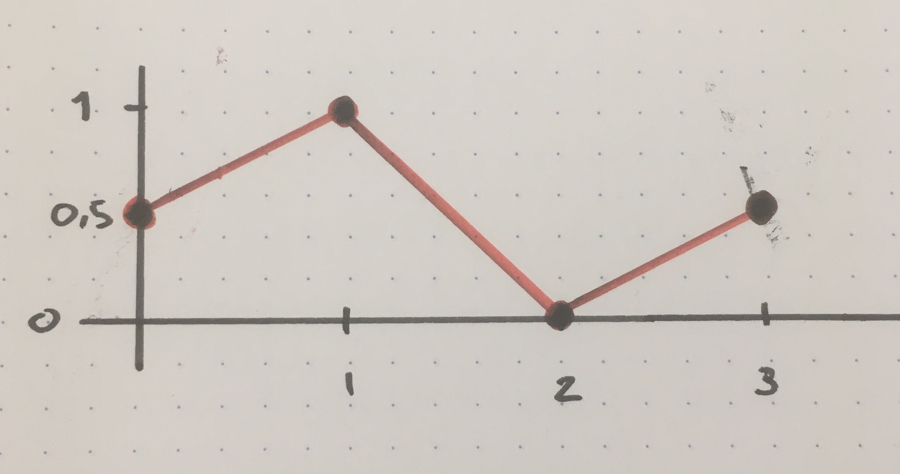Convert a list of points to a list of lines in Python

Do you need to create a poly line from a collection of points? This article shows you an example of how to use a recursive Python function to do that.

Let’s say you have a collection of four points like this:To draw lines between the points, you need to convert the four points to three lines like this:Expressed in numbers, you convert these points:

``````(0, 0.5)
(1, 1)
(2, 0)
(3, .5)
``````

to these lines:

``````(0, 0.5) -> (1, 1)
(1, 1) -> (2, 0)
(2, 0) -> (3, 0.5)
``````

Here is a recursive Python function that converts the list of points to a list of lines.

Create lines from points function

``````def create_lines_from_points(points, lines = []):
if len(points) == 0:
# no more points, return collected lines
return lines
else:
if len(lines) == 0:
if len(points) == 1:
raise ValueError('Cannot create line from one point')
# starting situation, return create_lines_from_points with args:
# 1: remaining points after first two points
# 2: line created from first two points
return create_lines_from_points(points[2:], [(points, points)])
else:
# mid-list situation, return create_lines_from_points with args:
# 1: remaining points
# 2: collected lines plus a new line based on one point from
#    the last line and a point from the list
return create_lines_from_points(points[1:], lines + [(lines[-1], points)])
``````

Usage:

``````points = [(0, 0.5), (1, 1), (2, 0), (3, 0.5)]
print(create_lines_from_points(points))
``````

Output:

``````[((0, 0.5), (1, 1)), ((1, 1), (2, 0)), ((2, 0), (3, 0.5))]
[Finished in 0.031s]
``````
Grüsse aus Berlin!
This is a cookie free and popup free website.Join me in making the web enjoyable again by removing the need for annoying cookie warnings and silly newsletter overlays!